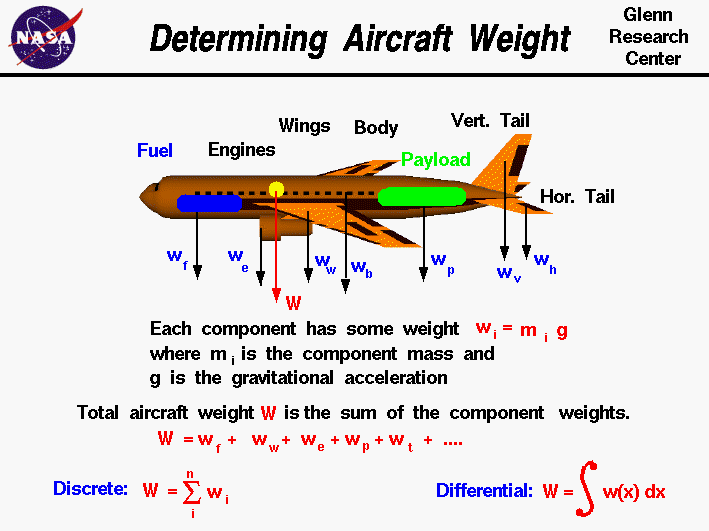+ Text Only Site
+ Non-Flash Version
+ Contact GlennWeight is the force generated by the gravitational attraction of the earth on the airplane. Each part of the aircraft has a unique weight and mass, and for some problems it is important to know the distribution. But for total aircraft maneuvering, we only need to be concerned with the total weight and the location of the center of gravity. The center of gravity is the average location of the mass of any object. How do engineers determine the weight of an airplane which they are designing? An airplane is a combination of many parts; the wings, engines, fuselage, and tail, plus the payload and the fuel. Each part has a weight associated with it which the engineer can estimate, or calculate, using Newton's weight equation: w = m * g where w is the weight, m is the mass, and g is the gravitational constant which is 32.2 ft/square sec in English units and 9.8 meters/square sec in metric units. The mass of an individual component can be calculated if we know the size of the component and its chemical composition. Every material (iron, plastic, aluminum, gasoline, etc.) has a unique density. Density r is defined to be the mass divided by the volume v: r = m / v If we can calculate the volume m = r * v The total weight W of the aircraft is simply the sum of the weight of all of the individual components. W = w(fuselage) + w(wing) + w(engines) + w(payload) + w(fuel) + ... To generalize, if we have a total of "n" discrete components, the weight of the aircraft is the sum of the individual i component weights with the index i going from 1 to n. The greek mathematical symbol sigma is used by mathematicians to denote this addition. (Sigma is a zig-zag symbol with the index designation being placed below the bottom bar, the total number of additions placed over the top bar, and the variable to be summed placed to the right of the sigma with each component designated by the index.) W = SUM(i=1 to i=n) [wi] This equation says that the weight of the airplane is equal to the sum of the weight of "n" discrete parts. What if the parts are not discrete? What if we had a continuous change of mass from front to back? The continuous change can be computed using integral calculus. The sigma designation is changed to the integral "S" shaped symbol to denote a continuous variation. W = S w(x) dx The discrete weight is replaced with w(x) which indicates that the weight is some function of distance x. If we are given the form of the function, there are methods to solve the integration. If we don't know the actual functional form, we can still numerically integrate the equation using a spread sheet by dividing the distance up into a number of small distance segments and determining the average value of the weight over that small segment, then summing up the value. Activities:Guided ToursAircraft Weight:Fuselage:Navigation ..Beginner's Guide Home Page+ Inspector General Hotline + Equal Employment Opportunity Data Posted Pursuant to the No Fear Act + Budgets, Strategic Plans and Accountability Reports + Freedom of Information Act + The President's Management Agenda + NASA Privacy Statement, Disclaimer, and Accessibility CertificationEditor: Nancy Hall NASA Official: Nancy Hall Last Updated: May 13 2021 + Contact Glenn